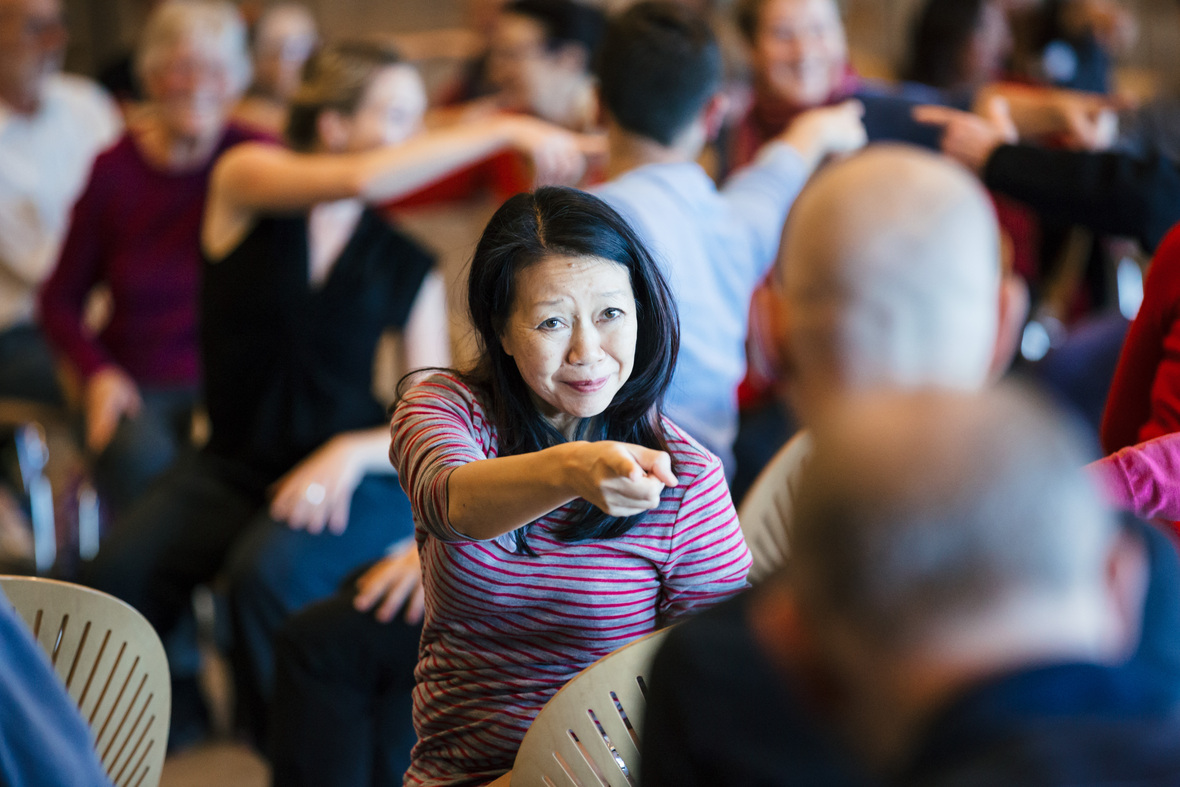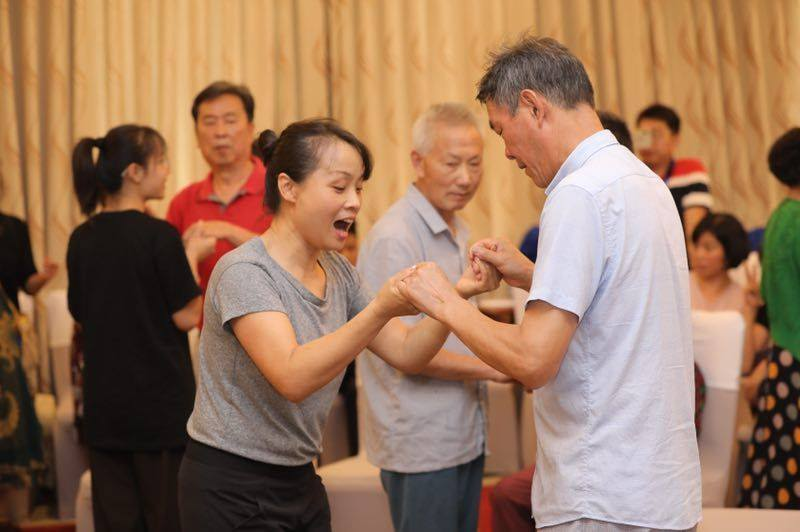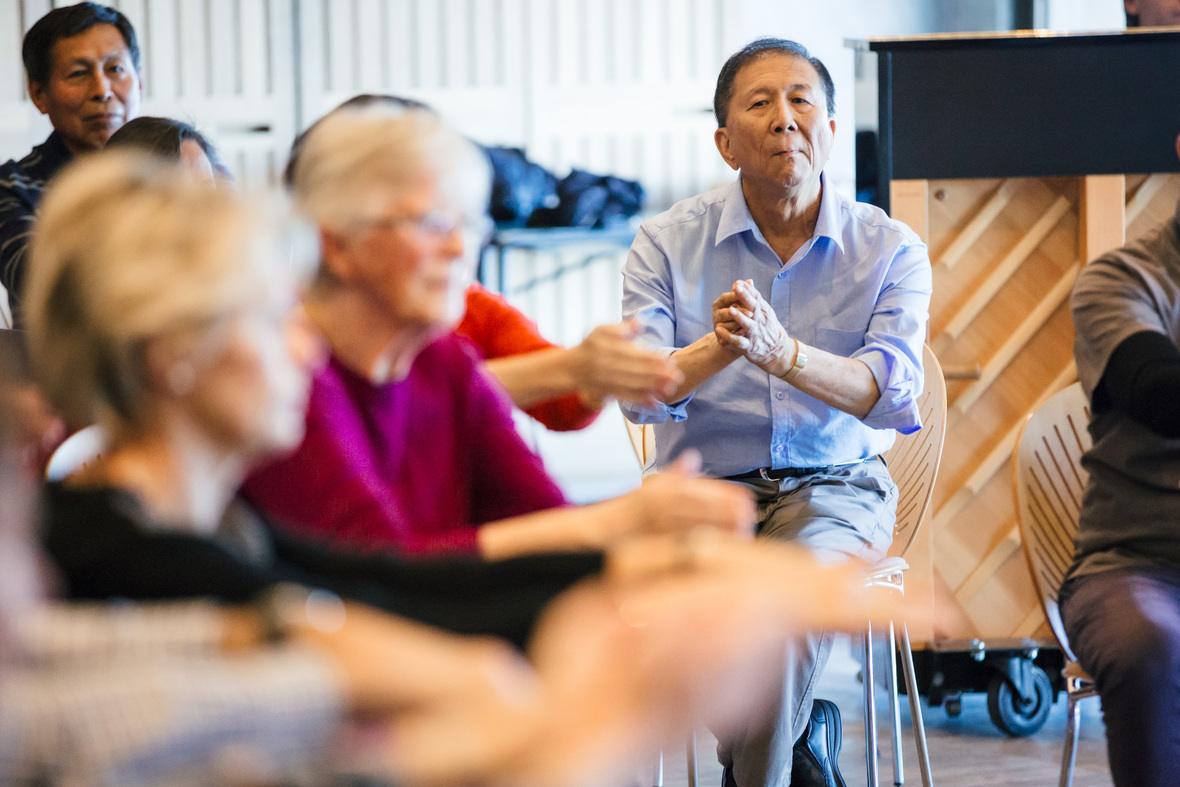Like   Tweet   +1
 table div table+table+table div table td,table.module-2{width:100%;padding:0}table div table+table+table div table{width:100%;float:none;margin-left:auto;margin-right:auto;padding:0}table div table+table+table div table a{border:0 none;text-decoration:none}table div table+table+table div table img{width:100%!important;border:0 none;text-decoration:none}/* styles */

# 2022 年春季舞动帕金森® 中英文双语版

## 为帕金森症患者，家人，朋友，以及护理人员量身订造的免费课程# 课程时间

## 8-9 AM （夏威夷檀香山）

 table.module-6{width:61.89%;padding:0}table div table+table+table+table+table+table+table div table{width:61.89%;float:none;margin-left:auto;margin-right:auto;padding:0}table div table+table+table+table+table+table+table div table a{border:0 none;text-decoration:none}table div table+table+table+table+table+table+table div table img{width:100%!important;border:0 none;text-decoration:none}table div table+table+table+table+table+table+table div table td{width:100%;padding:0}/* styles */
 /* styles */ ► 注册一次便可获有所有课程，您可以任意选择课程参与。注册后，您会通过电子邮件收到每周课程提醒及课程链接，每节课的访问信息都相同。 ► 在专业训练的老师的引导下，每节课开始会有一个简短的欢迎以及课程介绍环节，紧接着会有四十至五十分钟的舞蹈课。课程最后，我们诚邀所有参与者加入小组讨论并结识课友。 ► 在线课程将会在Zoom平台上实时提供，请点击此处了解如何在您的电脑及手机里下载Zoom软件。成功安装Zoom软件后，若您想设置默认语言为简体中文或繁体中文，请点击此处了解如何更改默认语言。若您有其他问题，我们的工作人员会在上课前十五分钟内为您解疑释惑。 ►作为我们提供尽可能安全环境的承诺的一部分，任何计划参加面对面课程的人都必须提供疫苗接种证明才能参加。此外，无论您疫苗接种状态，所有人员在室内必须戴口罩。 ►马克莫里斯舞团与纽约皇后剧院致力于社区参与，我们并不会拒绝任何人参与我们的课程。对此有任何疑问的人请联系maria@danceforpd.org。 ►我们的安全指南中对疫苗接种政策和口罩要求有详细概述。更多关于COVID-19安全信息可在我们的常见问题解答中找到。# 我们期待早日与您一起舞动！

 table div table+table+table+table+table+table+table+table+table+table+table div table{width:100%;padding:0}table div table+table+table+table+table+table+table+table+table+table+table div table img{width:96.23%;padding:0;float:none}table div table+table+table+table+table+table+table+table+table+table+table div table td{width:100%;padding:0 1.88% 18px}/* styles */table div table+table+table+table+table+table+table+table+table+table+table+table div table{width:100%;padding:0}table div table+table+table+table+table+table+table+table+table+table+table+table div table table{padding:0;float:left!important;width:43.584%!important}table div table+table+table+table+table+table+table+table+table+table+table+table div table table+table td,table div table+table+table+table+table+table+table+table+table+table+table+table div table td{padding-left:0;padding-right:0}table div table+table+table+table+table+table+table+table+table+table+table+table div table table td{padding-left:0;padding-right:20px}table div table+table+table+table+table+table+table+table+table+table+table+table div table table+table{float:left!important;width:56.416%!important}/* styles */
 table div table+table+table+table+table+table+table+table+table+table+table+table+table div table{width:100%;padding:0}table div table+table+table+table+table+table+table+table+table+table+table+table+table div table table{padding:0;float:left!important;width:50.188%!important}table div table+table+table+table+table+table+table+table+table+table+table+table+table div table table+table td,table div table+table+table+table+table+table+table+table+table+table+table+table+table div table td{padding-left:0;padding-right:0}table div table+table+table+table+table+table+table+table+table+table+table+table+table div table table td{padding-left:0;padding-right:20px}table div table+table+table+table+table+table+table+table+table+table+table+table+table div table table+table{float:left!important;width:49.812%!important}/* styles */
 /* styles */ 除了来自全球的热心个人支持者以外，舞动帕金森®课程还受利于 The Laurie M. Tisch Illumination Fund, The Parkinson’s Foundation, the American Parkinson Disease Association, Bossak/Heilbron Charitable Foundation, The Billy Rose Foundation, Boston Scientific Inc., Megara Foundation, New York City Council Member Helen Rosenthal, the New York City Department of Cultural Affairs, and the Zeitz Foundation的支持.